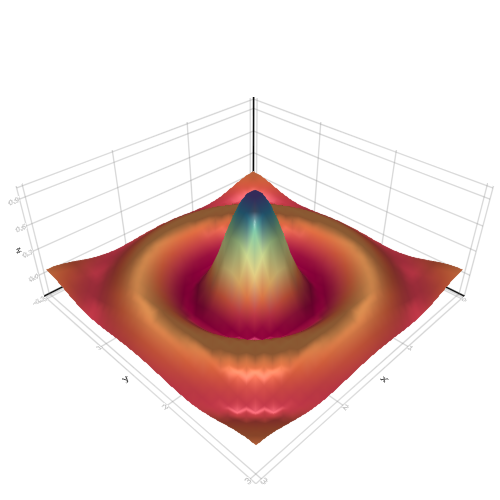## Surface

```

using AbstractPlotting

N = 30
function xy_data(x, y)
r = sqrt(x^2 + y^2)
r == 0.0 ? 1f0 : (sin(r)/r)
end
lspace = range(-10, stop = 10, length = N)
z = Float32[xy_data(x, y) for x in lspace, y in lspace]
r = range(0, stop = 3, length = N)
surface(
r, r, z,
colormap = :Spectral
)

```